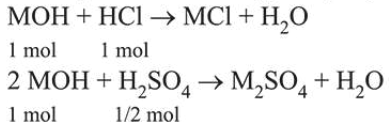# The first and second ionisation enthalpies of a metal are 496 and 4560 kJ`
Question:

The first and second ionisation enthalpies of a metal are 496 and $4560 \mathrm{~kJ} \mathrm{~mol}^{-1}$, respectively. How many moles of $\mathrm{HCl}$ and $\mathrm{H}_{2} \mathrm{SO}_{4}$, respectively, will be needed to react completely with 1 mole of the metal hydroxide?

1. 1 and 1

2. 2 and $0.5$

3. 1 and 2

4. 1 and $0.5$

Correct Option: , 4

Solution:

A large difference between first and second ionisation enthalpies $\left(4560-496=4064 \mathrm{~kJ} \mathrm{~mol}^{-1}\right)$ confirms the metal to be an alkali metal and thus is monovalent and form hydroxide of the type $\mathrm{M}(\mathrm{OH})$.